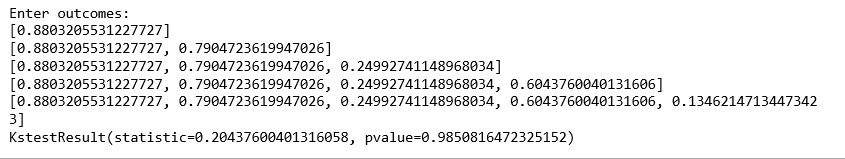ML | Kolmogorov-Smirnov Test – GeeksforGeeks

Kolmogorov–Smirnov test a very efficient way to determine if two samples are significantly different from each other. It is usually used to check the uniformity of random numbers. Uniformity is one of the most important properties of any random number generator and Kolmogorov–Smirnov test can be used to test it.
The Kolmogorov–Smirnov test may also be used to test whether two underlying one-dimensional probability distributions differ. It is a very efficient way to determine if two samples are significantly different from each other.

The Kolmogorov–Smirnov statistic quantifies a distance between the empirical distribution function of the sample and the cumulative distribution function of the reference distribution, or between the empirical distribution functions of two samples.

To use the test for checking the uniformity of random numbers, we use the CDF (Cumulative distribution function) of U[0, 1].

F(x)=x  for 0<=x<=1

Empirical CDF, Sn(x)= (number of R1, R2...Rn < x) / N array of random numbers, the random numbers must be in the range of [0, 1].

Hypothesis Used –

H0(Null Hypothesis): Null hypothesis assumes that the numbers are uniformly distributed between 0-1.
If we are able to reject the Null Hypothesis, this means that the numbers are not uniformly distributed between 0-1. Failure to reject the Null Hypothesis although does not necessarily mean that the numbers follow the uniform distribution.

kstest function in scipy Python –

Parameters:

Statistics: This is the calculated value of D, where D=|F(x)-Sn(x)|.
-> This D is compared with Dalpha where alpha is the level of significance. Alpha is defined as the probability of rejecting the null hypothesis given the null hypothesis(H0) is true. For most of the practical applications, alpha is chosen as 0.05.

p-value: This is calculated with the help of D.
-> If pvalue> alpha, we fail to reject the null hypothesis. Otherwise, we conclude that the numbers are not uniform. Ideally, the p-value should be as large as possible. For perfect uniform distribution pvalue=1 and Statisitics=0.

 from scipy.stats import kstest import random    N = 5    actual =[] print("Enter outcomes: ") for i in range(N):          actual.append(random.random())    print(actual) x = kstest(actual, "uniform")    print(x)

Output:KS Test is a very powerful way to automatically differentiate samples from a different distribution. kstest function may also be used to check whether the data given follows Normal Distribution or not. It compares the observed versus the expected cumulative relative frequencies of the Normal Distribution. The Kolmogorov-Smirnov test uses the maximal absolute difference between the observed and expected cumulative distribution.

• The Null hypothesis used here assumes that the numbers follow the normal distribution.
• The functioning of the function remains exactly same. Again it returns statistics and p-value. If the p-value is < alpha, we reject the Null hypothesis.
 from scipy.stats import kstest import random    N = 10    actual =[] print("Enter outcomes: ")    for i in range(N):          actual.append(random.random())    print(actual) x = kstest(actual, "norm")    print(x)

Output:If you like GeeksforGeeks and would like to contribute, you can also write an article using contribute.geeksforgeeks.org or mail your article to contribute@geeksforgeeks.org. See your article appearing on the GeeksforGeeks main page and help other Geeks.

Please Improve this article if you find anything incorrect by clicking on the "Improve Article" button below.

Article Tags :

Be the First to upvote.

Please write to us at contribute@geeksforgeeks.org to report any issue with the above content.